Computer Science‎ > ‎

## JOT_postEvent('registerForRpc', this, ['7554427248333330173', 1159382768, '//sfhp3v9mapo0ekuljg30ii29smp3u9co-a-sites-opensocial.googleusercontent.com/gadgets/ifr?url\x3dhttp://www.thelearningpoint.net/home/social.xml\x26container\x3denterprise\x26view\x3ddefault\x26lang\x3den\x26country\x3dALL\x26sanitize\x3d0\x26v\x3d77c64659765591c7\x26libs\x3dcore:dynamic-height\x26mid\x3d3\x26parent\x3dhttp://www.thelearningpoint.net/computer-science/python-for-data-2-simple-regression-with-matrix#up_if_twfollow_bool\x3dtrue\x26up_number_fbcomments\x3d4\x26up_if_inshare_bool\x3dtrue\x26up_if_pin_bool\x3dtrue\x26up_if_gplus_bool\x3dtrue\x26up_announce_page_url\x26up_if_tweet_bool\x3dtrue\x26up_if_custom_domain\x3dfalse\x26up_if_fbshare_bool\x3dtrue\x26up_via\x26st\x3de%3DAIHE3cDZgIM5dp1y2nxIdU0UpUV6nJSpObWcLnj3pd2BvnYZy0Sy4lawRPYptuduHbWvfqHJiOHuhxpLTT7miJHwC1T%252FhMUibPWOD80oWIcbq3u0Q3fERKrgneFW5tGdYnmKNNw1VgXm%26c%3Denterprise\x26rpctoken\x3d7554427248333330173'])

Simple Regression with Matrix
 Dear Folks, here we are gonna do simple linear regression, that means with numpy and matrix. Before going into this let's understand it by a simple example-Now we can predict our Y_hat for 3 (estimated y). Don't be stress if you don't understand these mathematics explanation read    this.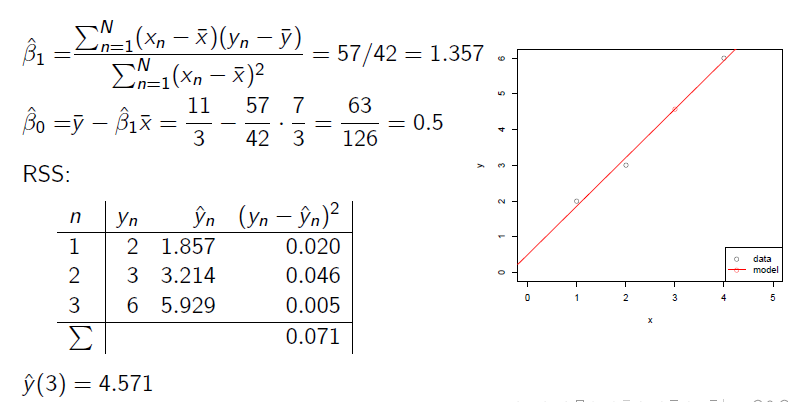Let's try it.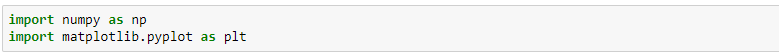Here numpy essential fom mathematical operations and matplotlib is to plot the graph of our linear model.Let's generate a simple data i.e. a matrix A with dimensions of 100x2. Initialize it with normal distributionmu = 2 and alpha = 0:01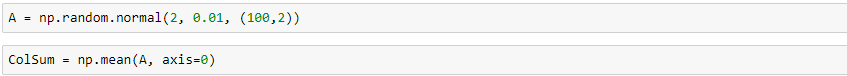here 'A' is a matrix with normal distribution mu = 2 & alpha = 0.01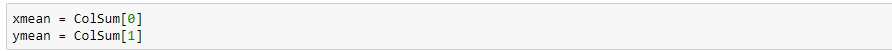Here we are defining x_bar and Y_bar (Xmean/Ymean). see the algorithm below.Here one can see that we need to follow above step to complete simple linear regression with matrix. Therefore, we are taking mean of X & Y.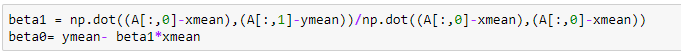Now we are going to calculate our betas (parameter).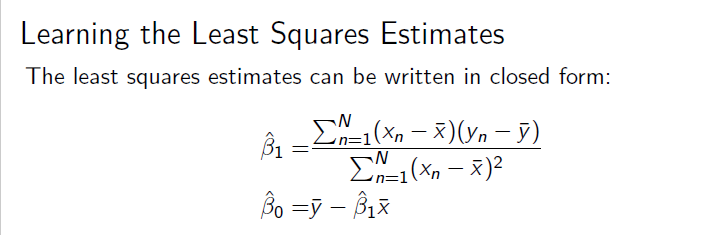This is how we calculate our betas (parameter). One can simply relate to the code and formula.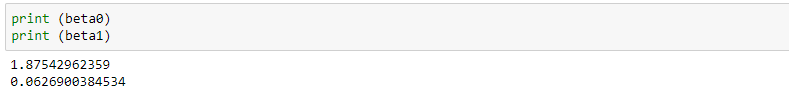this is our current beta. It can be different for you, since we are randomly initializing the data e.i. matrix.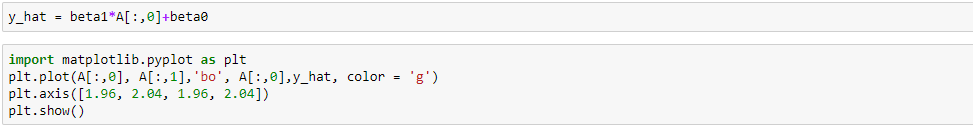Here we found out our prediction for ground truth e.t. Y_hat. Let's Plot the training points from matrix A and predicted values in the form of line graph.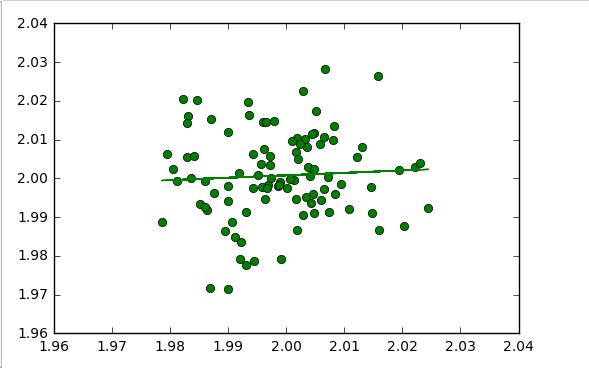At the end let's use numpy.linalg lstsq to replace step 2 for learning values of .Beta_0 and .Beta_1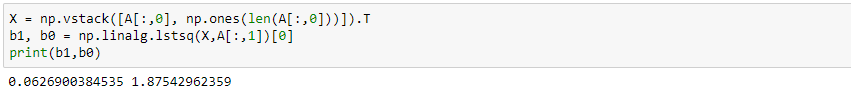here you can see that we have optimize the same beta as we did before manually. Hope It will help you all to understand simple regression better. What's Next 1) Logistic Regression2) Gradient Descent 3) Stochastic Gradient Descent